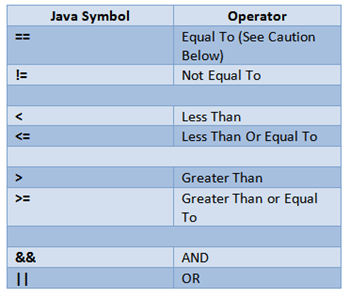MOCKSTACKS
EN# Java Operators

• An operator is a symbol that the compiler to perform a specific operation on operands.
• Example : a + b = c
• In the above example, 'a' and 'b' are operands on which the '+' operator is applied.

• ### Types of operators :

#### 1. Arithmetic Operators :

• Arithmetic operators are used to perform mathematical operations such as addition, division, etc on expressions.
• Arithmetic operators cannot work with Booleans.
• % operator can work on floats and doubles.
• Let x=7 and y=2

•#### 2. Comparison Operators :

• As the name suggests, these operators are used to compare two operands.
• Let x=7 and y=2

•#### 3.Logical Operators

• These operators determine the logic in an expression containing two or more values or variables.
• Let x = 8 and y =2

•#### 4. Bitwise Operators

• These operators perform the operations on every bit of a number.
• Let x =2 and y=3. So 2 in binary is 100, and 3 is 011.

•### Precedence of operators

The operators are applied and evaluated based on precedence. For example, (+, -) has less precedence compared to (*, /). Hence * and / are evaluated first. In case we like to change this order, we use parenthesis ().

### Example

``````package com.company;

public class CWH_Ch2_Operators {
public static void main(String[] args) {
// 1. Arithmetic Operators
int a = 4;
int b = 6 % a; // Modulo Operator
// 4.8%1.1 --> Returns Decimal Remainder

// 2. Assignment Operators
int b = 9;
b *= 3;
System.out.println(b);

// 3. Comparison Operators
System.out.println(64<6);

// 4. Logical Operators
System.out.println(64>5 && 64>98);
System.out.println(64>5 || 64>98);

// 5. Bitwise Operators
System.out.println(2&3);
//        10
//        11
//        ----
//        10
}
}
``````

## Conclusion

In this page (written and validated by ) you learned about Java Operators . What's Next? If you are interested in completing Java tutorial, your next topic will be learning about: Java Associativity of Operators.

Incorrect info or code snippet? We take very seriously the accuracy of the information provided on our website. We also make sure to test all snippets and examples provided for each section. If you find any incorrect information, please send us an email about the issue: mockstacks@gmail.com.